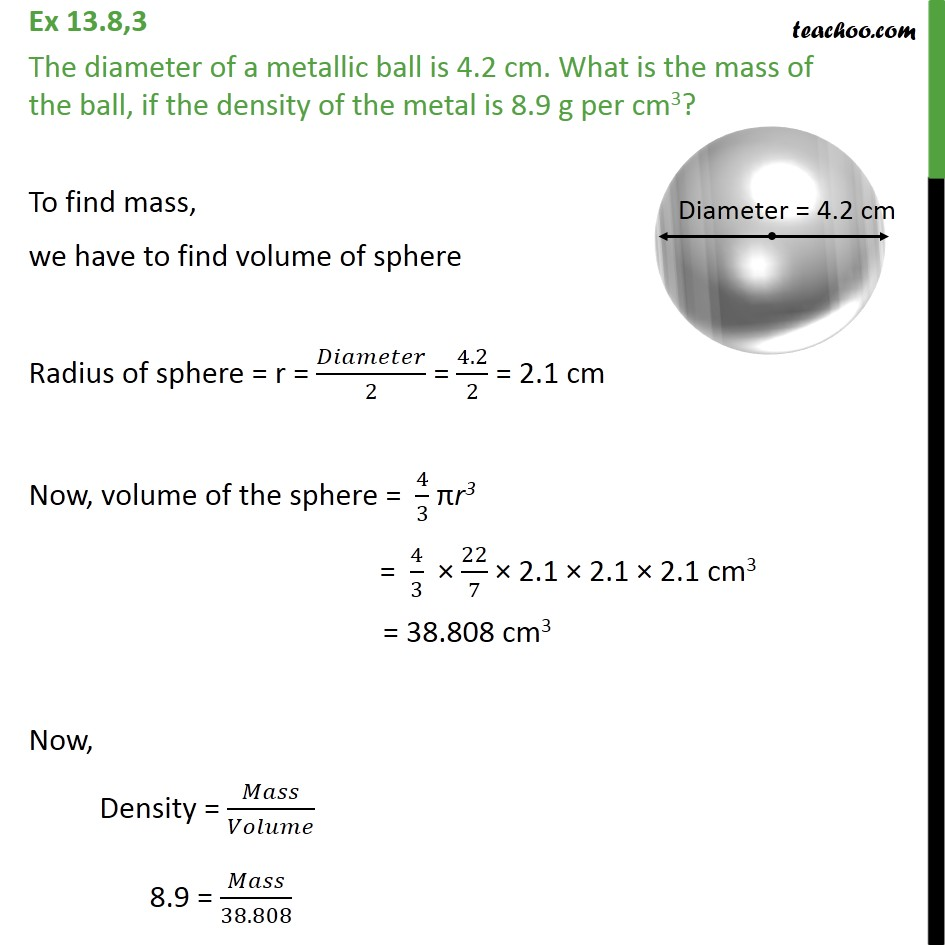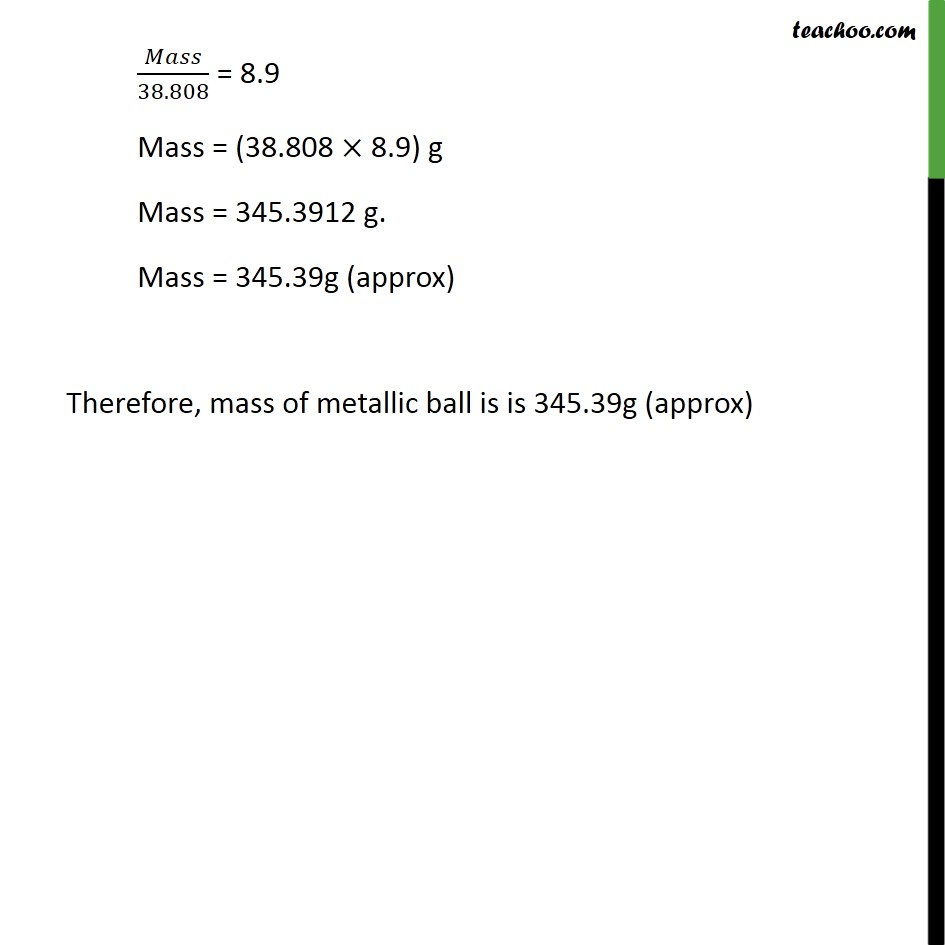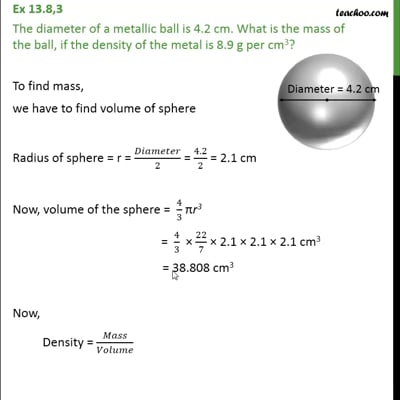Ex 13.8

Chapter 13 Class 9 Surface Areas and Volumes (Term 2)
Serial order wiseThis video is only available for Teachoo black users

### Transcript

Ex 13.8,3 The diameter of a metallic ball is 4.2 cm. What is the mass of the ball, if the density of the metal is 8.9 g per cm3? To find mass, we have to find volume of sphere Radius of sphere = r = 𝐷𝑖𝑎𝑚𝑒𝑡𝑒𝑟/2 = 4.2/2 = 2.1 cm Now, volume of the sphere = 4/3 πr3 = 4/3 × 22/7 × 2.1 × 2.1 × 2.1 cm3 = 38.808 cm3 Now, Density = 𝑀𝑎𝑠𝑠/𝑉𝑜𝑙𝑢𝑚𝑒 8.9 = 𝑀𝑎𝑠𝑠/38.808 𝑀𝑎𝑠𝑠/38.808 = 8.9 Mass = (38.808 × 8.9) g Mass = 345.3912 g. Mass = 345.39g (approx) Therefore, mass of metallic ball is is 345.39g (approx)